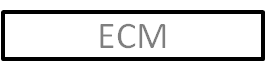# Evolving Clustering Method (ECM)## ECM Algorithm

This is based on the concept of dynamically adding and modifying the clusters as new data is presented, where the modification to the clusters affects both the position of the clusters and the size of the cluster, in terms of a radius parameter associated with each cluster that determines the boundaries of that cluster. ECM has only one parameter, which drives the addition of clusters, known as the distance threshold ${D}_{\mathrm{thr}}$. When new clusters are added, their centres are set to equal the example that triggered their creation, and the radius $R$of a new cluster is initially set to zero. $R$grows as more vectors are allocated to the cluster. Due to the mechanism by which $R$is updated, it cannot exceed ${D}_{\mathrm{thr}}$. The ECM algorithm is shown below:

Create the first cluster centre from the first example ${I}_{0}$

for each subsequent vector ${I}_{n}$do

Find the minimum distance ${D}_{min}$ between ${I}_{n}$ and each cluster centre ${C}_{n}$

if ${D}_{min}$ is less than any cluster radius then

Add ${I}_{n}$ to the nearest cluster

else

FInd the cluster $a$ with minimum value of ${S}_{i,j}$, where ${S}_{i,j}={D}_{i,j}+{R}_{i,j}$, ${D}_{i,j}$ is the distance between the cluster centre and vector $j$, and ${R}_{i}$ is the radius of cluster $i$

if ${S}_{i,a}>2{D}_{\mathrm{thr}}$ then

Create a new cluster

else

Update $a$

end if

end if

end for

When cluster $a$ is updated, its centre is shifted closer to ${I}_{n}$ and its radius ${R}_{a}\left(t+1\right)$ is set according to the equation below:

${R}_{a}\left(t+1\right)=\frac{{S}_{i,a}}{2}$

The new centre of $a,{C}_{a}\left(t+1\right)$ is set so that its distance is on the line between ${C}_{a}\left(t\right)$ and ${I}_{n}$ at a distance of ${R}_{a}\left(t+1\right).$

Maintained by Dr Michael J. Watts